International Patient Summary Implementation Guide
1.1.0 - CI BuildInternational Patient Summary Implementation Guide, published by HL7 International / Patient Care. This is not an authorized publication; it is the continuous build for version 1.1.0. This version is based on the current content of https://github.com/HL7/fhir-ips/ and changes regularly. See the Directory of published versions

## Data Type Profile: Ratio (IPS)

 Official URL: http://hl7.org/fhir/uv/ips/StructureDefinition/Ratio-uv-ips Version: 1.1.0 Standards status: Trial-use Maturity Level: 2 Computable Name: RatioIPS

Ratio data type, constrained to use UCUM as the code system for units.

Allow only UCUM as the code system for units.

Usage:

### Formal Views of Profile Content

This structure is derived from Ratio

NameFlagsCard.TypeDescription & Constraints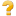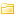Ratio 0..*RatioA ratio of two Quantity values - a numerator and a denominator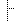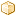numerator S0..1QuantityIPSA measured amount using UCUMdenominator S0..1QuantityIPSA measured amount using UCUMDocumentation for this format
NameFlagsCard.TypeDescription & ConstraintsRatio C0..*RatioA ratio of two Quantity values - a numerator and a denominatornumerator SC0..1QuantityIPSA measured amount using UCUMdenominator SC0..1QuantityIPSA measured amount using UCUMDocumentation for this format

#### Constraints

 Id Grade Path(s) Details Requirements ele-1 error **ALL** elements All FHIR elements must have a @value or children: hasValue() or (children().count() > id.count()) qty-3 error Ratio.numerator, Ratio.denominator If a code for the unit is present, the system SHALL also be present: code.empty() or system.exists() rat-1 error Ratio Numerator and denominator SHALL both be present, or both are absent. If both are absent, there SHALL be some extension present: (numerator.empty() xor denominator.exists()) and (numerator.exists() or extension.exists())
NameFlagsCard.TypeDescription & ConstraintsRatio C0..*RatioA ratio of two Quantity values - a numerator and a denominator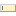id 0..1stringUnique id for inter-element referencingextension 0..*ExtensionAdditional content defined by implementations
Slice: Unordered, Open by value:urlnumerator SC0..1QuantityIPSA measured amount using UCUMDocumentation for this format

#### Constraints

 Id Grade Path(s) Details Requirements ele-1 error **ALL** elements All FHIR elements must have a @value or children: hasValue() or (children().count() > id.count()) ext-1 error **ALL** extensions Must have either extensions or value[x], not both: extension.exists() != value.exists() qty-3 error Ratio.numerator, Ratio.denominator If a code for the unit is present, the system SHALL also be present: code.empty() or system.exists() rat-1 error Ratio Numerator and denominator SHALL both be present, or both are absent. If both are absent, there SHALL be some extension present: (numerator.empty() xor denominator.exists()) and (numerator.exists() or extension.exists())

This structure is derived from Ratio

Summary

Must-Support: 2 elements

Structures

This structure refers to these other structures:

Maturity: 2

Differential View

This structure is derived from Ratio

NameFlagsCard.TypeDescription & ConstraintsRatio 0..*RatioA ratio of two Quantity values - a numerator and a denominatornumerator S0..1QuantityIPSA measured amount using UCUMdenominator S0..1QuantityIPSA measured amount using UCUMDocumentation for this format

Key Elements View

NameFlagsCard.TypeDescription & ConstraintsRatio C0..*RatioA ratio of two Quantity values - a numerator and a denominatornumerator SC0..1QuantityIPSA measured amount using UCUMdenominator SC0..1QuantityIPSA measured amount using UCUMDocumentation for this format

#### Constraints

 Id Grade Path(s) Details Requirements ele-1 error **ALL** elements All FHIR elements must have a @value or children: hasValue() or (children().count() > id.count()) qty-3 error Ratio.numerator, Ratio.denominator If a code for the unit is present, the system SHALL also be present: code.empty() or system.exists() rat-1 error Ratio Numerator and denominator SHALL both be present, or both are absent. If both are absent, there SHALL be some extension present: (numerator.empty() xor denominator.exists()) and (numerator.exists() or extension.exists())

Snapshot View

NameFlagsCard.TypeDescription & ConstraintsRatio C0..*RatioA ratio of two Quantity values - a numerator and a denominatorid 0..1stringUnique id for inter-element referencingextension 0..*ExtensionAdditional content defined by implementations
Slice: Unordered, Open by value:urlnumerator SC0..1QuantityIPSA measured amount using UCUMDocumentation for this format

#### Constraints

 Id Grade Path(s) Details Requirements ele-1 error **ALL** elements All FHIR elements must have a @value or children: hasValue() or (children().count() > id.count()) ext-1 error **ALL** extensions Must have either extensions or value[x], not both: extension.exists() != value.exists() qty-3 error Ratio.numerator, Ratio.denominator If a code for the unit is present, the system SHALL also be present: code.empty() or system.exists() rat-1 error Ratio Numerator and denominator SHALL both be present, or both are absent. If both are absent, there SHALL be some extension present: (numerator.empty() xor denominator.exists()) and (numerator.exists() or extension.exists())

This structure is derived from Ratio

Summary

Must-Support: 2 elements

Structures

This structure refers to these other structures:

Maturity: 2

Other representations of profile: CSV, Excel, Schematron